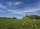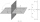# Quadratic equation + analytic geometry - math problems

#### Number of problems found: 24

• Sphere equationObtain the equation of sphere its centre on the line 3x+2z=0=4x-5y and passes through the points (0,-2,-4) and (2,-1,1).
• Equation of circle 2Find the equation of a circle which touches the axis of y at a distance 4 from the origin and cuts off an intercept of length 6 on the axis x.
• Distance problem 2A=(x,2x) B=(2x,1) Distance AB=√2, find value of x
• Curve and lineThe equation of a curve C is y=2x² -8x+9 and the equation of a line L is x+ y=3 (1) Find the x co-ordinates of the points of intersection of L and C. (2) Show that one of these points is also the stationary point of C?
• Intersections 3Find the intersections of the circles x2 + y2 + 6 x - 10 y + 9 = 0 and x2 + y2 + 18 x + 4 y + 21 = 0
• SupposeSuppose you know that the length of a line segment is 15, x2=6, y2=14 and x1= -3. Find the possible value of y1. Is there more than one possible answer? Why or why not?
• CircleFrom the equation of a circle: ? Calculate the coordinates of the center of the circle S[x0, y0] and radius of the circle r.
• Distance problemA=(x, x) B=(1,4) Distance AB=√5, find x;
• ProveProve that k1 and k2 are the equations of two circles. Find the equation of the line that passes through the centers of these circles. k1: x2+y2+2x+4y+1=0 k2: x2+y2-8x+6y+9=0
• On a lineOn a line p : 3 x - 4 y - 3 = 0, determine the point C equidistant from points A[4, 4] and B[7, 1].
• On lineOn line p: x = 4 + t, y = 3 + 2t, t is R, find point C, which has the same distance from points A [1,2] and B [-1,0].
• 3d vector componentThe vector u = (3.9, u3) and the length of the vector u is 12. What is is u3?
• Touch x-axisFind the equations of circles that pass through points A (-2; 4) and B (0; 2) and touch the x-axis.
• CircleThe circle touches two parallel lines p and q, and its center lies on line a, which is the secant of lines p and q. Write the equation of the circle and determine the coordinates of the center and radius. p: x-10 = 0 q: -x-19 = 0 a: 9x-4y+5 = 0
• Find parametersFind parameters of the circle in the plane - coordinates of center and radius: ?
• CircleWrite the equation of a circle that passes through the point [0,6] and touch the X-axis point [5,0]: ?
• CircleCircle is given by centre on S[-7; 10] and maximum chord 13 long. How many intersect points have circle with the coordinate axes?
• Find the 15Find the tangent line of the ellipse 9 x2 + 16 y2 = 144 that has the slope k = -1
• Tangents to ellipseFind the magnitude of the angle at which the ellipse x2 + 5 y2 = 5 is visible from the point P[5, 1] .
• Points in spaceThere are n points, of which no three lie on one line and no four lies on one plane. How many planes can be guided by these points? How many planes are there if there are five times more than the given points?

Do you have an interesting mathematical word problem that you can't solve it? Submit a math problem, and we can try to solve it.

We will send a solution to your e-mail address. Solved examples are also published here. Please enter the e-mail correctly and check whether you don't have a full mailbox.

Please do not submit problems from current active competitions such as Mathematical Olympiad, correspondence seminars etc...

For Basic calculations in analytic geometry is a helpful line slope calculator. From coordinates of two points in the plane it calculate slope, normal and parametric line equation(s), slope, directional angle, direction vector, the length of segment, intersections the coordinate axes etc. Looking for help with calculating roots of a quadratic equation? Quadratic Equations Problems. Analytic geometry - math word problems.